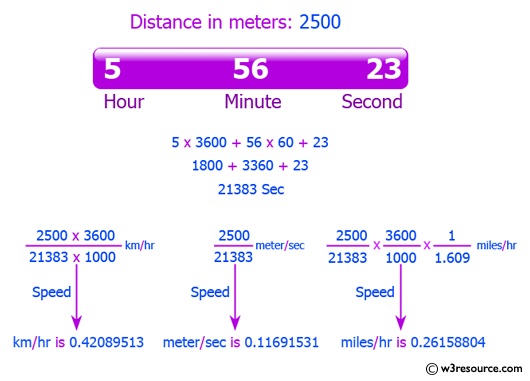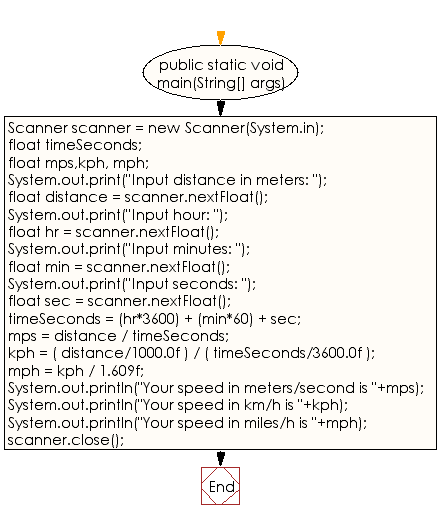﻿ Java exercises: Calculate speed in meters per second, kilometers and miles per hour - w3resource# Java Data Type Exercises: Calculate speed in meters per second, kilometers and miles per hour

## Java Data Type: Exercise-7 with Solution

Write a Java program to display the speed, in meters per second, kilometers per hour and miles per hour.
User Input : Distance (in meters) and the time was taken (as three numbers: hours, minutes, seconds).
Note : 1 mile = 1609 meters

Test Data
Input distance in meters: 2500
Input hour: 5
Input minutes: 56
Input seconds: 23Sample Solution:

Java Code:

``````import java.util.Scanner;
public class Exercise7 {

public static void main(String[] args) {
Scanner scanner = new Scanner(System.in);

float timeSeconds;
float mps,kph, mph;

System.out.print("Input distance in meters: ");
float distance = scanner.nextFloat();

System.out.print("Input hour: ");
float hr = scanner.nextFloat();

System.out.print("Input minutes: ");
float min = scanner.nextFloat();

System.out.print("Input seconds: ");
float sec = scanner.nextFloat();

timeSeconds = (hr*3600) + (min*60) + sec;
mps = distance / timeSeconds;
kph = ( distance/1000.0f ) / ( timeSeconds/3600.0f );
mph = kph / 1.609f;

System.out.println("Your speed in meters/second is "+mps);
System.out.println("Your speed in km/h is "+kph);
System.out.println("Your speed in miles/h is "+mph);

scanner.close();
}
}
```
```

Sample Output:

```Input distance in meters: 2500
Input hour: 5
Input minutes: 56
Input seconds: 23
Your speed in meters/second is 0.11691531
Your speed in km/h is 0.42089513
Your speed in miles/h is 0.26158804
```

Flowchart:Java Code Editor :

Improve this sample solution and post your code through Disqus

What is the difficulty level of this exercise?

﻿

## Java: Tips of the Day

Checks if a string is upper case:

```public static boolean isUpperCase(String input) {
return Objects.equals(input, input.toUpperCase());
}
```

Ref: https://bit.ly/39Hpo84